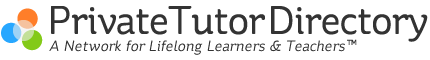Teaching Algebra Concepts to Young Learners
January 25, 2011 by Terry VanNoy  |

My students have told me often that in the upper elementary school years, 4th or 5th grade, they started to learn Algebra.  Usually, this memory evokes shudders of pain.  Some explain that they never really “got the hang of Algebra”, and that it looks like “just a bunch of letters, numbers, and mixed-up rules” jumbled together.  This has been a common discussion over the years with students who somehow got moved on from the comfortable levels of Arithmetic into the strange world of Algebra before they were really ready for it.  How young is too young for us to teach our students algebraic concepts?

The answer --- kids are never too young to learn algebra; they just need to be introduced to it in the right way, when they are ready.  So, if it looks like algebra is next in your child’s curriculum list, what do you do?

This question is actually part of the problem . . .  Algebra should not be handled as a separate unit at a certain time.  It is actually best taught as an embedded idea as soon as children know how to count and can use elementary mathematical symbols.  In short, if they can count, add and subtract, then they are ready.

Algebra quite simply is the study of arithmetic structure. . .  So, how does the teacher introduce algebra concepts to the young student?   Even at the youngest ages, our children can be ready for the following lesson sequence:

1.        Build on the arithmetic strategies the student knows already.  Introduce algebra ideas in a natural, comfortable way, linking from the natural, mathematical ideas of counting and basic numeric operations.  Discuss money exchanges, adding and subtracting objects from piles and groups.

Examples:
“If I have \$16 in my pocket and pay Shari, and I am left with \$9, how much did I pay her?”

“Jeff has three pieces of gum in one pocket and five in the other.  How many altogether?  If he has three in one pocket but eleven total, how many in this pocket . . .”

“Jeff has three pieces in this pile, as you can see, but 12 in total.  How many are in the pile I am covering?”

2.       Make it interactive and fun.  You want your student to be engaged and participate in the situations you present orally.  Use “mystery numbers” and unknown numbers of pieces as the stand-ins for variables.  Use funny sounds to represent the variables in a different order to create aural representations of equations.  Let your kids make up their own examples and create funny sounds.  Make it a hands-on experience whenever possible.

Examples:

“I am a number.  When I add 6 more, I have 13.  What number am I?”

“15 plus (oooomff) makes 28.  How much is (oooomff)”

“(arrrrrrgh) groups of 8 makes 32.  How many groups is (arrrrrrgh)?”

3.       Introduce rules and properties in the student’s own language, and then move into the proper math vocabulary of “rules of equality”, “equal operations”, and “distributive property”.

Use the concepts of fairness and balance.  You want the concepts to be learned before students are required to label the procedures formally.

To start talking about the concept of variables and equations, the learning sequence is extremely important here.  If the developmental stages are skipped, students will try to memorize procedures and the understanding is lost. Orally introduce situations where there is an unknown quantity or mystery number, then represent the variable a funny sound or gesture, then move on to written symbols.  Avoid using letter variables until your students are ready.

In summary, algebra is the study of the arithmetic structures of our world, and is a toolbox we use to solve problems.  The abstract nature of variables and equations and properties can only be understood after a proper developmental sequence of hands-on and oral examples, moving into the symbolic realm, and then progressing into pencil and paper exercises.  Make it fun and interactive; allow your students to create their own examples and tap into their own curiosity.  Embed these ideas into their basic curriculum lessons, not as a separate unit.  Students of algebra need to be able to see how variables and equations are used to do any type of problem solving, and are tools to unlock mysteries in the world of numbers and patterns.